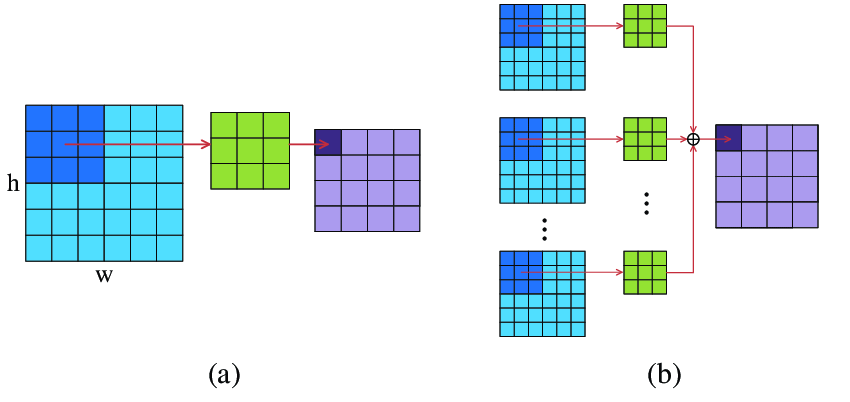# Dive into TensorIR

TensorIR is a compiler abstraction for optimizing programs with tensor computation primitives in TVM. Imagine a DNN task as a graph, where each node represents a tensor computation. TensorIR explains how each node/tensor computation primitive in the graph is carried out. This post explains my attempt to implement 2D convolution using TensorIR. It is derived from the Machine Learning Compilation course offered by Tianqi Chen.

## Implement 2D Convolution

2D convolution is a common operation in image processing. The image below captures how 2D convolution operates. I won’t go into details here. But you can find plenty information online regarding convolution.First, we initialize both the input matrix and the weight matrix:

# batch, input_channel_dim, image_height, image_width, output_channel_dim, kernel_width & height
N, CI, H, W, CO, K = 1, 1, 8, 8, 2, 3
# output_height, output_width, assuming kernel has stride=1 and padding=0
OUT_H, OUT_W = H - K + 1, W - K + 1
data = np.arange(N*CI*H*W).reshape(N, CI, H, W)
weight = np.arange(CO*CI*K*K).reshape(CO, CI, K, K)


We can validate the results using torch.nn.functional.conv2d() from PyTorch.

One thing Tianqi recommended for starters is to write the implementation first in numpy, and then translate the numpy implementation to TensorIR. I started my implementation directly from TensorIR, before totally getting confused. So here’s how I approach the problem.

First, and perhaps most importantly, you should figure out the accessing pattern of the output matrix, and gradually fill up the compute rules for each element in the output matrix. So, we know the output matrix has a shape of (N, CO, OUT_H, OUT_w) (which corresponds to batch, number of output channels, output height, and output width). The numpy loop will look like:

for b in np.arange(0, N):
for co in np.arange(0, CO):
for h in np.arange(0, OUT_H):
for w in np.arange(0, OUT_W):
Y[b, co, h, w] = 0


Here, we access element in the output matrix one by one and initialize each element to be 0. Next, we will try to figure out how to compute each element. We know each element in the output matrix is just the sum of element-wise multiplication of both the 2D convolutional kernel (1 by 3 by 3) and the corresponding area in the input matrix (1 by 3 by 3):

for b in np.arange(0, N):
for co in np.arange(0, CO):
for h in np.arange(0, OUT_H):
for w in np.arange(0, OUT_W):
# init to 0
Y[b, co, h, w] = 0
# 2d conv kernel
for ci in np.arange(0, CI):
for kh in np.arange(0, K):
for kw in np.arange(0, K):
# reduction
Y[b, co, h, w] += A[b, ci, h+kh, w+kw] * W[co, ci, kh, kw]


We can verify the function has the same output as torch.nn.functional.conv2d() from PyTorch.

The next part is to translate the numpy code into TensorIR. I won’t go into every the details of every single line here, but you can find all explanations from this note.

The nested loop can be encapsulated using T.grid() like this:

@tvm.script.ir_module
class MyConv:
@T.prim_func
def conv2d(data: T.Buffer[(N, CI, H, W), "int64"],
weight: T.Buffer[(CO, CI, K, K), "int64"],
result: T.Buffer[(N, CO, OUT_H, OUT_W), "int64"]):
T.func_attr({"global_symbol": "conv2d", "tir.noalias": True})
# loop through each elem in the output matrix
for b, o, h, w in T.grid(N, CO, OUT_H, OUT_W):
# kernel access pattern
for kc, kh, kw in T.grid(CI, K, K):


Next, we define the block (a basic unit of computation in TensorIR). A block contains a set of block axes (vi, vj, vk) and computations defined around them. Here, we define the property about each block axes:

class MyConv:
@T.prim_func
def conv2d(data: T.Buffer[(N, CI, H, W), "int64"],
weight: T.Buffer[(CO, CI, K, K), "int64"],
result: T.Buffer[(N, CO, OUT_H, OUT_W), "int64"]):
T.func_attr({"global_symbol": "conv2d", "tir.noalias": True})
# impl
for b, o, h, w in T.grid(N, CO, OUT_H, OUT_W):
for kc, kh, kw in T.grid(CI, K, K):
with T.block("A"):
vb = T.axis.spatial(N, b)
vc_o = T.axis.spatial(CO, o)
vh = T.axis.spatial(OUT_H, h)
vw = T.axis.spatial(OUT_W, w)
vc_i = T.axis.reduce(CI, kc)
vw_h = T.axis.reduce(K, kh)
vw_w = T.axis.reduce(K, kw)


The outer loop all receives T.axis.spatial(), because we access each element in the output matrix element by element (spatially), without doing anything else. On the other hand, we see parameters in the innter loop receives T.axis.reduce(). Remember, each element in the output matrix is just the sum of element-wise multiplication of both the 2D convolutional kernel (1 by 3 by 3) and the corresponding area in the input matrix (1 by 3 by 3). Therefore, after the element-wise multiplication finishes, we need perform a reduction operation over all three axes. More concretely, we will sum up all elements in the row(K), column(K), and channel(CI): (1, 3, 3) -> (1)

@tvm.script.ir_module
class MyConv:
@T.prim_func
def conv2d(data: T.Buffer[(N, CI, H, W), "int64"],
weight: T.Buffer[(CO, CI, K, K), "int64"],
result: T.Buffer[(N, CO, OUT_H, OUT_W), "int64"]):
T.func_attr({"global_symbol": "conv2d", "tir.noalias": True})
# impl
for b, o, h, w in T.grid(N, CO, OUT_H, OUT_W):
for kc, kh, kw in T.grid(CI, K, K):
with T.block("A"):
vb = T.axis.spatial(N, b)
vc_o = T.axis.spatial(CO, o)
vh = T.axis.spatial(OUT_H, h)
vw = T.axis.spatial(OUT_W, w)
vc_i = T.axis.reduce(CI, kc)
vw_h = T.axis.reduce(K, kh)
vw_w = T.axis.reduce(K, kw)

with T.init():
result[vb, vc_o, vh, vw] = T.int64(0)
# compute rule
result[vb, vc_o, vh, vw] += data[vb, vc_i, vh+vw_h, vw+vw_w] * weight[vc_o, vc_i, vw_h, vw_w]